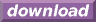#Calcute: freeware calculator

## Arcsecant (arc-secant, inverse secant, asec)

The arc-secant is the inverse of the secant function. Calcute implements it with the asec function. The inverse secant of a value x is the value y for which the secant of y is x. In other words if y = asec(x) then x = sec(y). And since the secant takes an angle as input parameter, the arcsecant produces an angle as its output.

```asec(-1)
3.14159265359
```

The asec value is expressed by Calcute using the currently-selected angle unit: radian, degree or gradient. In the above example, the result is in radians.

The arc-secant function is undefined in the open range (-1, +1); this matches the output domain of the secant function. Note also that the secant function is periodic: its values repeat infinitely for angles that represent more than one complete rotation. Conversely, the arcsecant is a multi-valued function: any parameter value matches an infinite number of possible angles. But the asec function generates a single value refered to as the principal value, chosen by convention with positive range [0, pi]. All other possibilities can be obtained by adding or subtracting any whole number of rotations from the return value of asec.

In some mathematical texts and hand-held calculators, the notation sec-1 is sometimes used to represent the arc-secant function. This is an unfortunate notational choice since it could also stand for the multiplicative inverse 1/sec, which is a different function.

Yet another term also used to represent the inverse secant function is "arcsec".